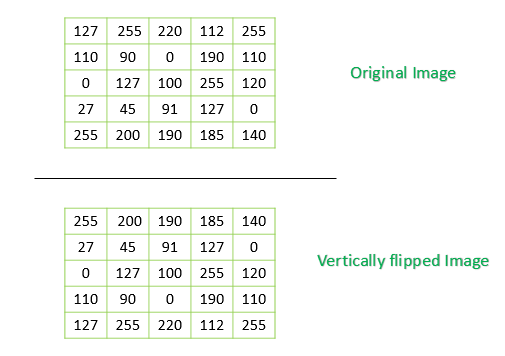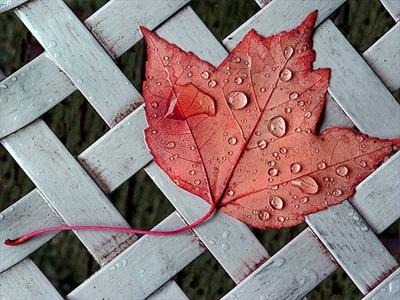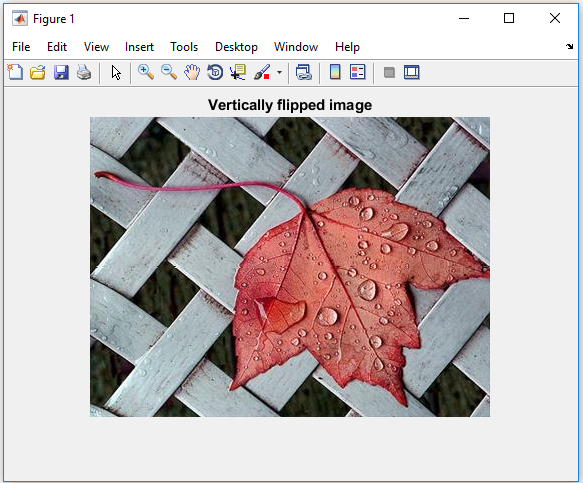Related Articles

# How to vertically flip an Image using MATLAB

• Last Updated : 14 Jan, 2020

Prerequisite: Image representation in MATLAB

In MATLAB, Images are stored in matrices, in which each element of the matrix corresponds to a single discrete pixel of the image. We can flip the given image vertically (along the x-axis), if we reverse the order of the pixels (elements of the matrix) in each column as illustrated in the below image.Code #1: Using MATLAB Library function

 `% Read the target image file``img = imread(``'leaf.png'``);``  ` `% Reverse the order of the element in each column``vertFlip_img = flip(img, 1);``  ` `% Display the vertically flipped image``imshow(vertFlip_img); ``title(``'Vertically flipped image'``);`

Code #2: Using matrix manipulation

 `% Read the target image file``img = imread(``'leaf.png'``);``  ` `% Flip the columns vertically ``vertFlip_img = img(``end` `: -1: 1, :, :);``  ` `% Display the vertically flipped image``imshow(vertFlip_img); ``title(``'Vertically flipped image'``);`

Code #3: Using matrix manipulation (Using loops)

Approach:

• Read the source image file in MATLAB environment
• Get the Dimensions of the image matrix
• Reverse the order of the elements of each column in every image plane
• Display the water image (vertically flipped image).

Below is the implementation of the above approach:

 `% Read the target image file``img = imread(``'leaf.png'``);``  ` `% Get the dimensions of the image``[x, y, z] = size(img);``  ` `  ` `% Reverse elements of each column``% in each image plane (dimension)``for` `plane = 1 : z``    ``len = x;``    ``for` `i = 1 : x ``        ``for` `j = 1 : y``  ` `            ``% To reverse the order of the element``            ``% of a column we can swap the ``            ``% topmost element of the row with``            ``% its bottom-most element  ``             ` `            ``if` `i < x/2 ``                ``temp = img(i, j, plane);``                ``img(i, j, plane) = img(len, j, plane);``                ``img(len, j, plane) = temp;``                ` `            ``end``        ``end``        ``len = len - 1;``    ``end``end``  ` `  ` `% Display the vertically flipped image``imshow(img); ``title(``'Vertically flipped image'``);`

Input image: `leaf.png`Output:My Personal Notes arrow_drop_up